• 用C++实现的一元线性回归数据处理，输入两组数据，可以输出斜率、截距和回归系数以及最终结果
• 一元线性回归测试数据，主要是含有一个属性值。通过这个属性值来预测输出。
• 2.1一元线性回归python代码 2.2sklearn代码 3示例 3.1波士顿房价预测 3.2北京房价预测 1.概览 2代码实现 2.1一元线性回归python代码 # -*- coding: utf-8 -*- """ Created on Wed Sep 19 ...

目录
1.概览
2代码实现
2.1一元线性回归python代码
2.2sklearn代码
3示例
3.1波士顿房价预测
3.2北京房价预测

1.概览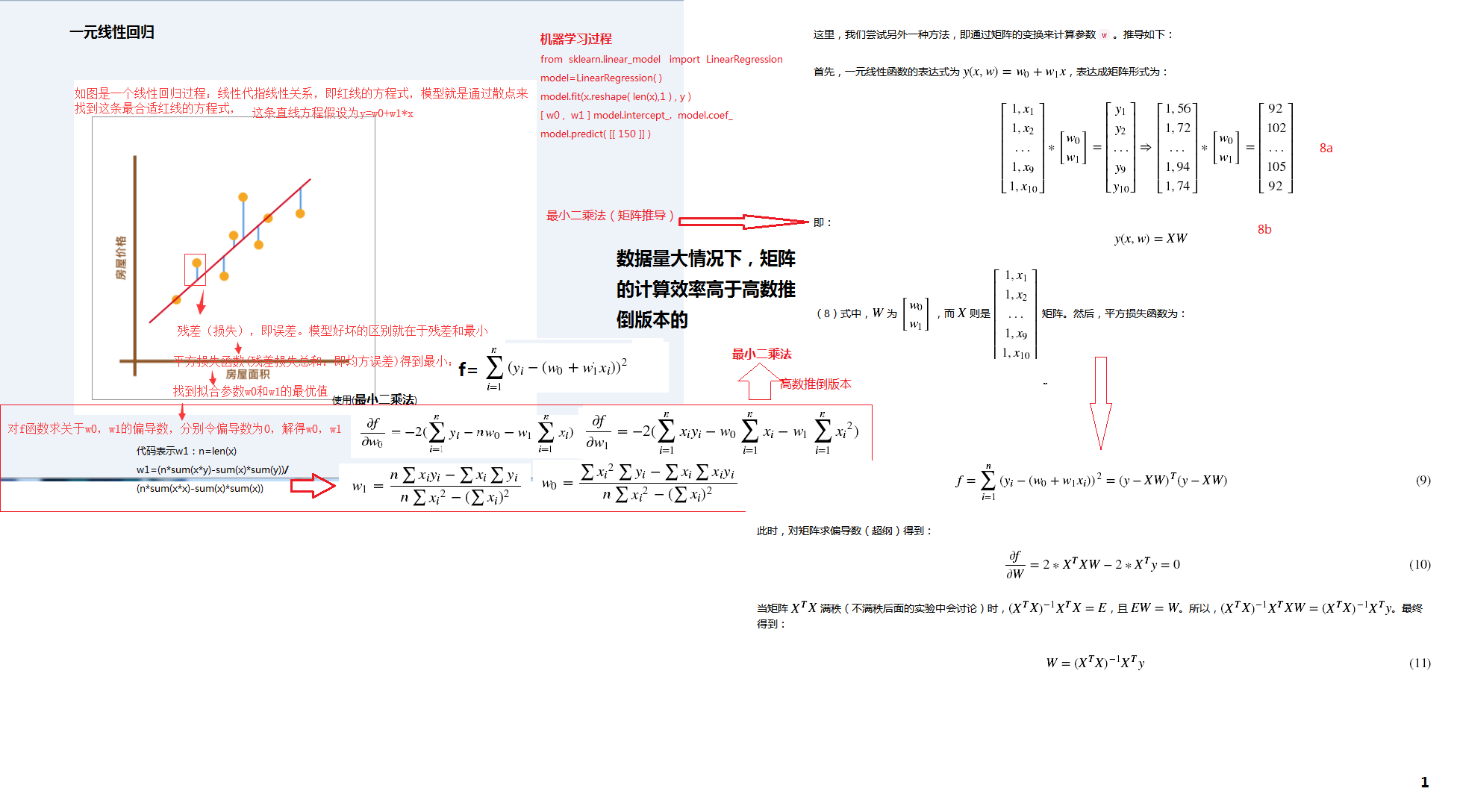2代码实现
2.1一元线性回归python代码
# -*- coding: utf-8 -*-
"""
Created on Wed Sep 19 11:12:27 2018
一元线性回归
数据：一组房屋面积和房价变化的示例数据。x 为房屋面积，单位是平方米; y 为房价，单位是万元。
"""
import numpy as np
x = np.array([56, 72, 69, 88, 102, 86, 76, 79, 94, 74])
y = np.array([92, 102, 86, 110, 130, 99, 96, 102, 105, 92])
"""一元函数式代码实现
"""
def f(x, w0, w1):
y = w0 + w1 * x
return y
"""平方损失函数实现
"""
def square_loss(x, y, w0, w1):
loss = sum(np.square(y - (w0 + w1*x)))
return loss
"""最小二乘法代数求解平方损失函数最小值
"""
def w_calculator(x, y):
n = len(x)
w1 = (n*sum(x*y) - sum(x)*sum(y))/(n*sum(x*x) - sum(x)*sum(x))
w0 = (sum(x*x)*sum(y) - sum(x)*sum(x*y))/(n*sum(x*x)-sum(x)*sum(x))
return w0, w1       #(41.33509168550616, 0.7545842753077117)
w0 = w_calculator(x, y)
w1 = w_calculator(x, y)
"""拟合结果画图展示
"""
x_temp = np.linspace(50,120,100) # 绘制直线生成的临时点
plt.scatter(x, y)
plt.plot(x_temp, x_temp*w1 + w0, 'r')
2.2sklearn代码
# -*- coding: utf-8 -*-
"""
Created on Wed Sep 19 11:38:20 2018
scikit-learn 线性回归拟合
"""
import numpy as np
x = np.array([56, 72, 69, 88, 102, 86, 76, 79, 94, 74])
y = np.array([92, 102, 86, 110, 130, 99, 96, 102, 105, 92])
from sklearn.linear_model import LinearRegression
# 定义线性回归模型
model = LinearRegression()
model.fit(x.reshape(len(x),1) , y) # 训练, reshape 操作把数据处理成 fit 能接受的形状
# 得到模型拟合参数
model.intercept_, model.coef_
print(model.predict([]))#[154.52273298]
3示例
3.1波士顿房价预测
# -*- coding: utf-8 -*-
"""
Created on Wed Sep 19 11:44:25 2018

波士顿房价预测
数据集共计 506 条，其中包含有 13 个与房价相关的特征以及 1 个目标值（房价）。
http://labfile.oss.aliyuncs.com/courses/1081/course-5-boston.csv
"""
import pandas as pd
features = df[['crim', 'rm', 'lstat']]
target = df['medv'] # 目标值数据
#分割
split_num = int(len(features)*0.7) # 得到 70% 位置
train_x = features[:split_num] # 训练集特征
train_y = target[:split_num] # 训练集目标
test_x = features[split_num:] # 测试集特征
test_y = target[split_num:] # 测试集目标
#建模
from sklearn.linear_model import LinearRegression
model = LinearRegression() # 建立模型
model.fit(train_x, train_y) # 训练模型
model.coef_, model.intercept_ # 输出训练后的模型参数和截距项
preds = model.predict(test_x) # 输入测试集特征进行预测
preds # 预测结果
"""误差计算
平均绝对误差（MAE）
均方误差（MSE）
"""
def mae_value(y_true, y_pred):
n = len(y_true)
mae = sum(np.abs(y_true - y_pred))/n
return mae
def mse_value(y_true, y_pred):
n = len(y_true)
mse = sum(np.square(y_true - y_pred))/n
return mse
mae = mae_value(test_y.values, preds)
mse = mse_value(test_y.values, preds)
print("MAE: ", mae)
print("MSE: ", mse)
输出：
MAE:  13.022063072780165 MSE:  303.8331247223573
3.2北京房价预测
# -*- coding: utf-8 -*-
"""
Created on Wed Sep 19 11:54:49 2018
北京市住房价格预测
http://labfile.oss.aliyuncs.com/courses/1081/challenge-1-beijing.csv
"""

"""读取并预览数据集
"""
import pandas as pd
"""将原始数据集切片为所需数据集
"""
features = df[['公交','写字楼','医院','商场','地铁','学校','建造时间','楼层','面积']]
target = df['每平米价格']
"""方法二
"""
#features = df[df.columns.drop(['小区名字','房型','每平米价格'])]
#target = df['每平米价格']
"""分割
"""
split_num = int(len(df)*0.7) # 70% 分割数
train_x = features[:split_num]
train_y = target[:split_num]
test_x = features[split_num:]
test_y = target[split_num:]
#建模
from sklearn.linear_model import LinearRegression
model = LinearRegression()
model.fit(train_x, train_y)
"""平均绝对百分比误差  MAPE
"""
import numpy as np
def mape(y_true, y_pred):
"""
参数:
y_true -- 测试集目标真实值
y_pred -- 测试集目标预测值
返回:
mape -- MAPE 评价指标
"""
n = len(y_true)
mape = 100 * np.sum(np.abs((y_true-y_pred)/y_true)) / n
return mape
y_true = test_y.values
y_pred = model.predict(test_x)
print(mape(y_true, y_pred))
"""
MAPEMAPE  值较大，意味着预测的偏移量较大。在线性回归实验中，

我们提到预测结果较差的一个原因可能是数据没有经过预处理。除此之外，线性回归本身就是一种非常基础简单的预测方法。
对于房价这种包含多个特征的预测问题，我们往往要使用更复杂的方法来进行回归预测才能得到更好的结果。
"""

展开全文回归算法
• 一元线性回归的Python代码测试数据，主要是包含一个属性值和label的测试数据
• 一元线性回归和多元线性回归表面意思容易理解，但是结合实际的数据集，会混乱。这也是在编写线性回归博文的时候梳理知识点发现自己的不足，同时记录下来，让有疑问的同学也可以得到答案，拨开乌云。 1.在数据集上的...
      一元线性回归和多元线性回归表面意思容易理解，但是结合实际的数据集，会混乱。这也是在编写线性回归博文的时候梳理知识点发现自己的不足，同时记录下来，让有疑问的同学也可以得到答案，拨开乌云。
1.在数据集上的异同
一元线性回归：
给定数据集，其中，样本有1个属性描述。
VS
多元线性回归：
给定数据集，其中 ，，样本有d个属性描述。

2.向量表达式
一元线性回归：

VS
多元线性回归：

展开全文• MATLAB 一元线性回归 含例题数据，和可运行的MATLAB代码
• 本文是从一元线性回归为为基础来理解线性回归，适合于线性回归的入门，让初学者对于线性回归有直观的理解。本文也是我对于线性回归算法入门学习，分享给大家。 线性回归的定义 回归是用于应用于输入变量与输出...
      本文是从一元线性回归为为基础来理解线性回归，适合于线性回归的入门，让初学者对于线性回归有直观的理解。本文也是我对于线性回归算法入门学习，分享给大家。
线性回归的定义
回归是应用于表达输入变量与输出变量的关系，在输入变量与输出变量之间做一个映射，一般的线性回归公式为

其中θ为权重参数，现在给定的数据是给定一些X与其对应的Y值，需要求解一组θ,将X映射到Y，在无误差的情况下，用n组已知的X与Y数据，用解方程的方式就可以解出θ。由于误差的存在，用n组数据解出的一组θ并不满足剩于组的数据，并且会一些矛盾解。现在将问题转为如何求解一组θ,让X最优映射到Y。下面从最简单的一元线性回归来理解说明回归的思想，如果一元线性回归的原理理解了，多维线性回归就理解了。
一元线性回归
一元线性回归，表示只有一个因变量，从数学公式来说就是一条直线，数学公式：

我们线性的生成100组数据，给定，a,b的，将产生的值叠加噪声，现在通过这100组值，来求解出让 与a,b接近。下面是程序生的100数据，后面所有的计算都是基于这100组数据产生的。
import numpy as np
import matplotlib.pyplot as plt
from mpl_toolkits.mplot3d import Axes3D
from numpy import *
sampleSize = 100
mu = 0
sigma = 0.01
np.random.seed(0)
g = np.random.normal(mu, sigma, sampleSize)
x = np.linspace(0,1,100)
a = 0.5
b = 0.5
y = x*a + b
print y
y2 = y + g
print y2
plt.plot(x, y)
plt.scatter(x, y2, color='red')
plt.grid()
plt.title("linear regression")
plt.show()
数据的图像：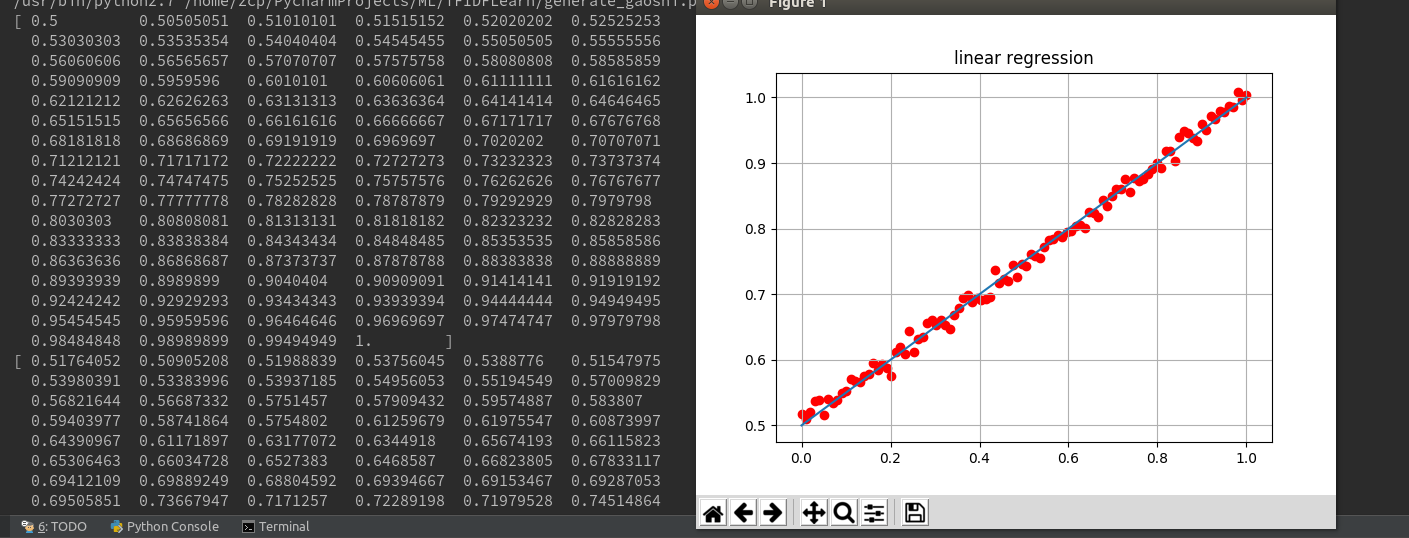从图上可以看出，点是分布在直线的两边，直线相当于是这些点的线性拟合，我们通过点来解求出这样的一点直线。给出这些点，我们可以画出很多直线，这时候我们就需要一个度量标准，来评判那条直线的优劣，这个标准也就是我们平时说的目标函数。我们经常采用的是误差平方和来作为目标函数，误差平方和越小，我们认为求出的越接近于理想值。数据表达式为：
我们用程序来实现，画出图形便于理解
theta0 = np.linspace(-20,20,100)
theta1 = np.linspace(-20,20,100)
J_theta0_theta1 = np.linspace(-1, 1, 100)
for i, t0 in enumerate(theta0):
t1 = theta1[i]
sum = 0
for j,xj in enumerate(x):
sum = sum + (t1*xj+t0-y2[j])*(t1*xj+t0-y2[j])
J_theta0_theta1[i] = sum*0.5
print J_theta0_theta1

figure = plt.figure()

ax = Axes3D(figure)
ax.scatter(theta0, theta1, J_theta0_theta1,color='r')

sum_x = np.sum(x)
sum_x2 = np.dot(x, x.T)
sum_y = np.sum(y2)
sum_y2 = np.dot(y2,y2.T)
sum_xy = np.sum(np.multiply(x,y))
print sum_xy
theta0, theta1 = np.meshgrid(theta0, theta1)
#z = sum_x2*np.dot(theta1, theta1.T) + 2*sum_x*np.dot(theta0,theta1.T) - 2 * sum_x * sum_y * theta1 - 2 * sum_y * theta0 + np.dot(theta0,theta0.T)
#print z
#z1 = np.dot(x, theta1.T) + theta0.T - y2.T
z = np.empty_like(theta0)
def computeCost(X, y2, t0, t1):
sum = 0
for j, xj in enumerate(X):
sum = sum + (t1 * xj + t0 - y2[j]) * (t1 * xj + t0 - y2[j])
return sum*0.5

for (i, j) ,v in np.ndenumerate(z):
z[i,j] = computeCost(x, y2, theta0[i, j], theta1[i, j])
print z
ax.plot_surface(theta0, theta1, z, rstride=1, cstride=1, cmap='rainbow')
ax.set_zlabel('J(theta0,theta1)')
ax.set_ylabel('theta1')
ax.set_xlabel('theta0')
plt.contour(theta0, theta1, z, 20, alpha=.75, cmap=plt.cm.hot)
#plt.contourf(theta0, theta1, z)
plt.show()
得到结果：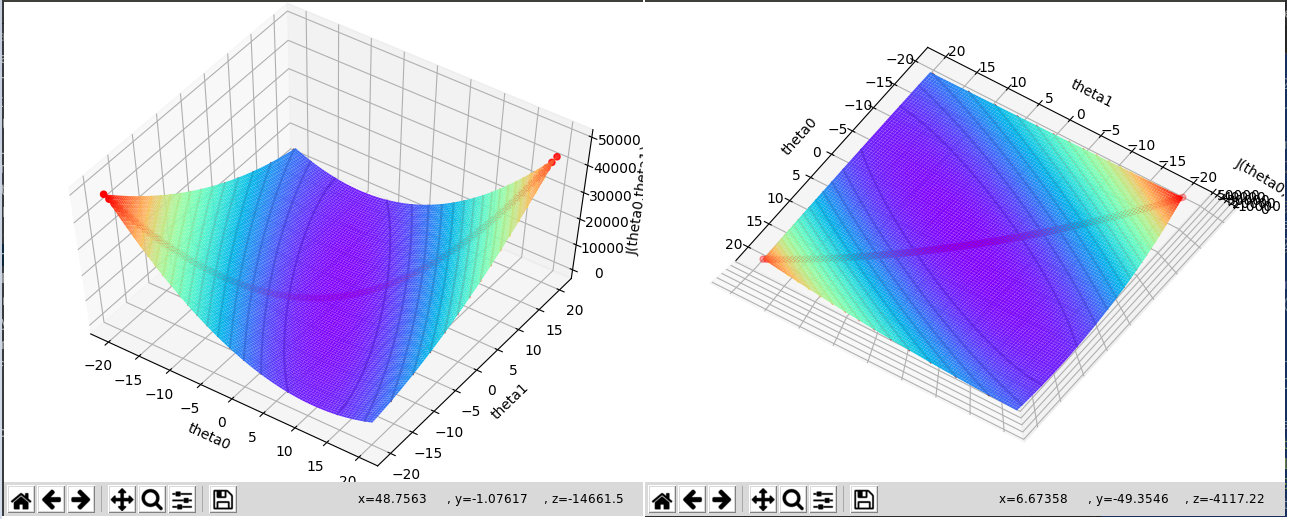目录函数据我们画了对角线上的点跟等高线，从这个图上可以看出，有一个极值点，极值点也就是最小点。求极值的方式就是让
求导让导数为0的点:

接上面程序，求解一元线性方程：
from scipy.linalg import solve

print "theta1,theta0", solve([[sum_x,100],[sum_x2,sum_x]],[sum_y,sum_xy])
解出结果为：theta1,theta0 [ 0.49648258 0.50235679] ，这样就能通过直接求的方式得到所需的参数。
目标函数的矩阵形式
结合上面部分，结合矩阵知识对公式进一步推导：

从公式上看，我们可以直接求出θ的值，当然为了防止过拟合，会加入扰动因子，这部分就不在这讨论了。
梯度下降算法的实现
上面的一元线性回归中，对于目标函数，对于任意给定的 ,如果沿着它们的负梯度方向衰减，衰减的步长足够小，就可以得到接近函数极值的点。对于一元线性回归的部分

程序部分：
# 利用梯度下降算法求解 theta0 theta1 初始值为1

# 迭代阀值，当两次迭代损失函数之差小于该阀值时停止迭代
epsilon = 0.0001

# 学习率
alpha = 0.001

theta0 = 20
theta1 = 1

n = len(x)
print "n=",n
J_theta = [0, 0]
iter_times = 0
g_x = []
g_y = []
g_z = []
while True:
diff = [0, 0]

for i in range(n):
diff += (theta0*1 + theta1*x[i] - y2[i])*1
diff += (theta0*1 + theta1*x[i] - y2[i])*x[i]

theta0 -= alpha * diff
theta1 -= alpha * diff
g_x.append(theta0)
g_y.append(theta1)

J_theta = 0
for i in range(n):
J_theta += (theta0*1 + theta1*x[i] - y2[i])**2

J_theta = 0.5 * J_theta
g_z.append(J_theta)
print "iter times %d ,theta0 = %s, theta1= %s, epsilon = %s " % (iter_times,theta0,theta1,abs(J_theta - J_theta))
if abs(J_theta - J_theta) < epsilon:
break
else:
J_theta = J_theta
iter_times += 1
print "theta0,theta1", theta0,theta1
ax.plot(g_x,g_y,g_z,c='black')
plt.show()
结果图：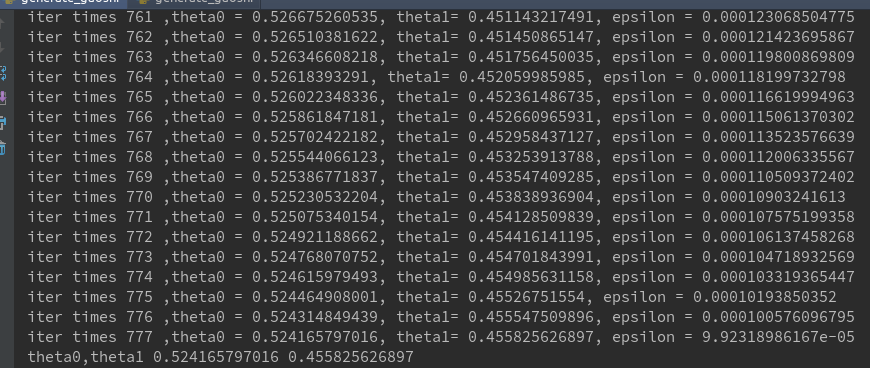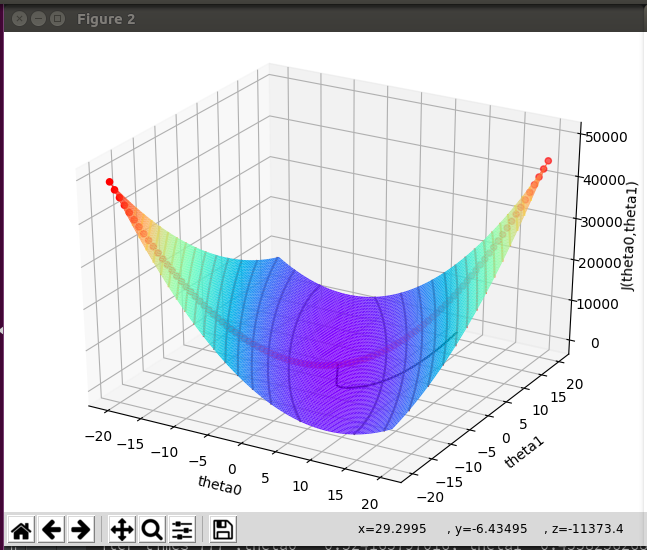当然我们可以调整不同参数，看运行的效果，图中的黑线是变化情况，最终我们也可得到一个近似的解，可以看出并不是我们上面直接解方程的解。
总结
理解了一元的线性回归，其实按照一元的线性回归的思想，就比较容易理多元的线性回归，并且我们看到随机梯度算法，批量梯度算法，mini-batch,都是梯度算法改进,梯度下降算法理解了，这些理解起来也比较容易了。本文只是回归的入门知识，如有不足，欢迎指正。
展开全文机器学习 梯度下降
• 问题制作一元材积表，不懂林学的可能不知道，如图，也就是构造材积和胸径间的关系，这里采用了python的一元线性回归方法（本人用spss做了幂函数非线性回归，效果最好）。Python方差分析导入库和数据from sklearn ...
问题
制作一元材积表，不懂林学的可能不知道，如图，也就是构造材积和胸径间的关系，这里采用了python的一元线性回归方法（本人用spss做了幂函数非线性回归，效果最好）。Python方差分析
导入库和数据
from sklearn import linear_model
import numpy as np
import pandas as pd
import matplotlib.pyplot as plt
绘制散点图
X = np.array(df1[['胸径']])
Y = np.array(df1[['材积']])
plt.rc('font',family='STXihei',size=15)
plt.scatter(X,Y,60,color='blue',marker='o',linewidths=3,alpha=0.4)
plt.xlabel('胸径')
plt.ylabel('材积')
plt.title('一元材积表')
plt.show()可以看出，用一元线性回归是不太理想的，不过为了给老师交作业，还是做一下好了。
一元回归模型
clf = linear_model.LinearRegression()
clf.fit(X,Y)
print(clf.coef_,clf.intercept_)
print(clf.score(X,Y))
结果如图结论
R2不高，模型并不太好。
展开全文• Python一元线性回归数据分析数据拆分数据建模模型保存模型调用 数据分析 import pandas as pd import numpy as np import matplotlib.pyplot as plt from pandas import DataFrame, Series from sklearpython
• 一元线性回归模型很简单y1=ax+b+ε，y1为实际值，ε为正态的误差。y2=ax+b，y2为预测值。ε=y1-y2。def model(a,b,x):# x is vector，a and b are the common number.return a*x+b这里将整组数据的预测结果方差作为...
• 无论时代再如何变，人类的知识体系永远不会变，除非这个世界本身就不是真实的。...找了许许多多的方案，最终决定尝试一种方案，一天下来没做啥，真的累了，数据更直观，用相应的公式和数据进行分...
• （MATLAB）一元线性回归和多元线性回归1.一元线性回归2.多元线性回归2.1数据说明2.2程序运行结果 1.一元线性回归 直接看代码，目标是建立yyy和xxx的函数关系，即求y=kx+by=kx+by=kx+b中的kkk和bbb，kkk和bbb都是实数...matlab 机器学习 人工智能
• 普通最小二乘估计对数据进行一元线性回归分析原理，附详细推导
• 一元线性回归模型 线性回归模型是数据科学领域最简单的模型，很多复杂的模型 （如多项式回归、逻辑回归、SVM） 都是建立在线性回归模型的基础上的，或者是从其中能找到线性回归模型的影子。最简单的线性回归模型就是...损失函数 机器学习 最小二乘法
• 回归分析中，如果有两个或两个以上的自变量，就称为多元回归。事实上，一种现象常常是与多个因素相联系的，由多个自变量的最优组合共同来预测或估计因变量，比只用一个自变量进行预测或估计更有效，更符合实际。 ...matlab 多元线性回归
• 一元线性回归 1、女士的身高-体重例子。--借助excel数据分析功能 2、气温-冰红茶销售量例子。--直接计算 多元线性回归 3、薪资-性别-年龄-教育程度例子。--借助excel数据分析功能 4、店铺营业额-店铺面积-离车站...机器学习
• 在 Jupyter Notebook 上用 Python 实现的一元线性回归，包括源码和数据，大家可以学习参考使用
• 一元线性回归一元线性回归模型一元线性回归的参数估计最小二乘估计最大似然估计最大似然估计数学推导有偏估计与无偏估计参数估计的性质一元线性回归的显著性检验一元线性回归的残差分析一元线性回归模型的应用 一元...
• 基于spss的一元线性回归与多元线性回归案例，个人整理出的，包含了部分案例、实验报告、题目，及部分题目答案，适合作为spss、MATLAB等软件数据分析题目联系
• 数据挖掘中的一元线性回归简单演示程序，利用了c#winform制作的界面，一目了然，对于数据挖掘初学者有很好的指导作用，一元线性回归的算法其实不难。
• 文章目录原理以及公式【1】一元线性回归问题【2】多元线性回归问题【3】学习率【4】流程分析（一元线性回归）【5】流程分析（多元线性回归）归一化原理以及每种归一化适用的场合一元线性回归代码以及可视化结果多元...可视化 python 深度学习 机器学习
• 解析法实现一元线性回归 代码： #加载样本数据 x=[137.97,104.50,100.00,124.32,79.20,99.00,124.00,114.00,106.69,138.05,53.75,46.91,68.00,63.02,81.26,86.21] y=[145.00,110.00,93.00,116.00,65.32,104.00,118....可视化 python 机器学习 numpy
• Jupyter nodebook工具做的简单的一元线性回归。将文件导入nodebook运行即可 数据摘自深入浅出数据分析这本书。这里用python3实现书中的R代码。有需要的学习python3进行数据分析的同学自行倒腾
• 回归分析中，只包括一个自变量和一个因变量，且二者的关系可用一条直线近似表示，这种回归分析称为一元线性回归分析。如果回归分析中包括两个或两个以上的自变量，且因变量和自变量之间是线性关...
• 来自烟水暖的学习笔记回归分析(Regression analysis)回归...回归分析的分类：1） 按自变量的个数，可以分为一元回归，多元回归2）按变量相关性的形状（回归线）是否为直线型，可分为线性回归，非线性回归。下面，......